# User:Tohline/H Book

(Difference between revisions)
 Revision as of 20:37, 27 February 2015 (view source)Tohline (Talk | contribs) (→Structure:: Relocate position of free-energy plot on the page)← Older edit Revision as of 13:10, 28 February 2015 (view source)Tohline (Talk | contribs) (→Spherically Symmetric Configurations: More word-smithing)Newer edit → Line 38: Line 38: ===Structure:=== ===Structure:=== - Here we show how the set of principal governing equations (PGEs) may be solved to determine the equilibrium structure of spherically symmetric fluid configurations — such as individual, nonrotating stars.  After supplementing the PGEs by specifying an equation of state of the fluid, the system of equations is usually solved by employing one of three techniques to obtain a "detailed force-balanced" model that provides the radius, $~R_\mathrm{eq}$, of the equilibrium configuration — given its mass, $~M$, and central pressure, $~P_c$, for example — as well as details regarding the internal radial profiles of the mass-density and fluid pressure.  As our various discussions illustrate, simply varying the power-law index, $~n$, in a ''polytropic'' equation of state, + Here we show how the set of principal governing equations (PGEs) may be solved to determine the equilibrium structure of spherically symmetric fluid configurations — such as individual, nonrotating stars.  After supplementing the PGEs by specifying an equation of state of the fluid, the system of equations is usually solved by employing one of three techniques to obtain a "detailed force-balanced" model that provides the radius, $~R_\mathrm{eq}$, of the equilibrium configuration — given its mass, $~M$, and central pressure, $~P_c$, for example — as well as details regarding the internal radial profiles of the fluid density and fluid pressure.  As our various discussions illustrate, simply varying the power-law index, $~n$, in a ''polytropic'' equation of state,
Line 89: Line 89: - where all three of the dimensionless ''structural form factors'', $~\mathfrak{f}_M$, $~\mathfrak{f}_W$, and $~\mathfrak{f}_A$, are generically ''of order unity'' for detailed force-balanced models having a wide range of internal structures and are all three exactly equal to unity under the assumption that the equilibrium configuration has uniform density and uniform pressure throughout.  Alternatively, if the polytropic constant, $~K$ (which specifies the specific entropy of fluid elements throughout the system), instead of the central pressure, is held fixed while searching for extrema in the free-energy, the virial equilibrium relation for isolated polytropes is, + where all three of the dimensionless ''structural form factors'', $~\mathfrak{f}_M$, $~\mathfrak{f}_W$, and $~\mathfrak{f}_A$, are unity, under the assumption that the equilibrium configuration has uniform density and uniform pressure throughout, and are otherwise generically ''of order unity'' for detailed force-balanced models having a wide range of internal structures.  Alternatively, if the specific entropy of fluid elements (set by the value of $~K$) throughout the system, rather than the central pressure, is held fixed while searching for extrema in the free-energy, the virial equilibrium relation for isolated polytropes is,
Line 95: Line 95:
- $~\biggl( \frac{G}{K} \biggr)^n M^{n-1} R_\mathrm{eq}^{3-n}$ + $~M^{(n-1)/n} R_\mathrm{eq}^{(3-n)/n} \biggl( \frac{G}{K} \biggr)$ Line 101: Line 101: - $~\frac{3}{4\pi} \biggl(\frac{5\mathfrak{f}_A \mathfrak{f}_M}{\mathfrak{f}_W} \biggr)^n \frac{1}{\mathfrak{f}_M} \, . + [itex]~\frac{5\mathfrak{f}_A \mathfrak{f}_M}{\mathfrak{f}_W} \biggl(\frac{3}{4\pi \mathfrak{f}_M}\biggr)^{1/n} \, .$ [/itex]
Line 107: Line 107: - If the physical system under consideration — such as a protostellar gas cloud — is not isolated but is, instead, embedded in a hot, tenuous medium that exerts on the system a confining external pressure, $~P_e$, the configuration's equilibrium parameters will be related via the expression, If the physical system under consideration — such as a protostellar gas cloud — is not isolated but is, instead, embedded in a hot, tenuous medium that exerts on the system a confining external pressure, $~P_e$, the configuration's equilibrium parameters will be related via the expression, Line 140: Line 139:
- $~K \biggl( \frac{3M}{4\pi R_\mathrm{eq}^3} \biggr)^{(n+1)/n} \cdot \frac{\mathfrak{f}_A}{\mathfrak{f}_M^{(n+1)/n}} + [itex]~K \mathfrak{f}_A \biggl( \frac{3M}{4\pi R_\mathrm{eq}^3} \cdot \frac{1}{\mathfrak{f}_M} \biggr)^{1 + 1/n} - - \biggl(\frac{3GM^2}{20\pi R_\mathrm{eq}^4} \biggr)\cdot \frac{\mathfrak{f}_W}{\mathfrak{f}^2_M} \, .$ + - \frac{\mathfrak{f}_W}{5} \biggl(\frac{3GM^2}{4\pi R_\mathrm{eq}^4} \cdot \frac{1}{\mathfrak{f}_M^2} \biggr) \, .[/itex]
- It is a virial expression specifically of this form that identifies extrema (e.g., valleys or ridges) in the rainbow-colored free-energy surface, $\mathfrak{G}^*(R_\mathrm{eq}, P_e)$, displayed above. + It is a virial expression specifically of this form $~($with $n = \infty$ and $\mathfrak{f}_M = \mathfrak{f}_W = \mathfrak{f}_A = 1)$ that identifies extrema (e.g., valleys or ridges) in the rainbow-colored free-energy surface, $\mathfrak{G}^*(R_\mathrm{eq}, P_e)$, displayed above.

## Revision as of 13:10, 28 February 2015

Preface from the original version of this HyperText Book (H_Book):

November 18, 1994

Much of our present, basic understanding of the structure, stability, and dynamical evolution of individual stars, short-period binary star systems, and the gaseous disks that are associated with numerous types of stellar systems (including galaxies) is derived from an examination of the behavior of a specific set of coupled, partial differential equations. These equations — most of which also are heavily utilized in studies of continuum flows in terrestrial environments — are thought to govern the underlying physics of all macroscopic "fluid" systems in astronomy. Although relatively simple in form, they prove to be very rich in nature... <more>

# Applications

## Spherically Symmetric Configurations

### Structure:

Here we show how the set of principal governing equations (PGEs) may be solved to determine the equilibrium structure of spherically symmetric fluid configurations — such as individual, nonrotating stars. After supplementing the PGEs by specifying an equation of state of the fluid, the system of equations is usually solved by employing one of three techniques to obtain a "detailed force-balanced" model that provides the radius,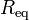$~R_\mathrm{eq}$, of the equilibrium configuration — given its mass,$~M$, and central pressure,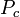$~P_c$, for example — as well as details regarding the internal radial profiles of the fluid density and fluid pressure. As our various discussions illustrate, simply varying the power-law index,$~n$, in a polytropic equation of state,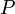$~P$$~=$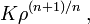$~K \rho^{(n+1)/n} \, ,$

gives rise to equilibrium configurations that have a wide variety of internal structural profiles.

If one is not particularly concerned about details regarding the distribution of matter within the equilibrium configuration, a good estimate of the size of the equilibrium system can be determined by assuming a uniform-density structure then identifying local extrema in the system's global free energy. An illustrative, undulating free-energy surface is displayed here, on the right; blue dots identify equilibria associated with a "valley" of the free-energy surface while white dots identify equilibria that lie along a "ridge" in the free-energy surface.

In the astrophysics community, the mathematical relation that serves to define the properties of configurations that are associated with such free-energy extrema is often referred to as the scalar virial theorem. Specifically, for isolated systems in virial equilibrium, the following relation between configuration parameters holds: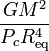$~\frac{GM^2}{P_c R_\mathrm{eq}^4}$$~=$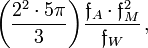$~\biggl(\frac{2^2\cdot 5 \pi}{3} \biggr) \frac{\mathfrak{f}_A \cdot \mathfrak{f}_M^2}{\mathfrak{f}_W} \, ,$

where all three of the dimensionless structural form factors,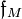$~\mathfrak{f}_M$,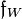$~\mathfrak{f}_W$, and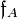$~\mathfrak{f}_A$, are unity, under the assumption that the equilibrium configuration has uniform density and uniform pressure throughout, and are otherwise generically of order unity for detailed force-balanced models having a wide range of internal structures. Alternatively, if the specific entropy of fluid elements (set by the value of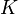$~K$) throughout the system, rather than the central pressure, is held fixed while searching for extrema in the free-energy, the virial equilibrium relation for isolated polytropes is,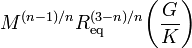$~M^{(n-1)/n} R_\mathrm{eq}^{(3-n)/n} \biggl( \frac{G}{K} \biggr)$$~=$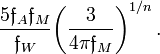$~\frac{5\mathfrak{f}_A \mathfrak{f}_M}{\mathfrak{f}_W} \biggl(\frac{3}{4\pi \mathfrak{f}_M}\biggr)^{1/n} \, .$

If the physical system under consideration — such as a protostellar gas cloud — is not isolated but is, instead, embedded in a hot, tenuous medium that exerts on the system a confining external pressure,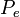$~P_e$, the configuration's equilibrium parameters will be related via the expression,$~\frac{GM^2}{P_c R_\mathrm{eq}^4}$$~=$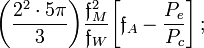$~\biggl(\frac{2^2\cdot 5 \pi}{3} \biggr) \frac{\mathfrak{f}_M^2}{\mathfrak{f}_W} \biggl[ \mathfrak{f}_A - \frac{P_e}{P_c} \biggr] \, ;$

or, fixing$~K$ instead of$~P_c$, the relevant virial equilibrium expression is,$~P_e$$~=$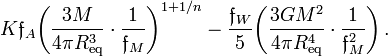$~K \mathfrak{f}_A \biggl( \frac{3M}{4\pi R_\mathrm{eq}^3} \cdot \frac{1}{\mathfrak{f}_M} \biggr)^{1 + 1/n} - \frac{\mathfrak{f}_W}{5} \biggl(\frac{3GM^2}{4\pi R_\mathrm{eq}^4} \cdot \frac{1}{\mathfrak{f}_M^2} \biggr) \, .$

It is a virial expression specifically of this form$~($with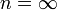$n = \infty$ and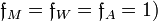$\mathfrak{f}_M = \mathfrak{f}_W = \mathfrak{f}_A = 1)$ that identifies extrema (e.g., valleys or ridges) in the rainbow-colored free-energy surface,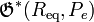$\mathfrak{G}^*(R_\mathrm{eq}, P_e)$, displayed above.

Solution Strategies:

Detailed Force-Balance
(Introduction)

Virial Equilibrium
(Introduction)

 Example Solutions: Uniform-density sphere Isolated … ✓ ✓ Embedded in an External Medium … ✓ ✓ Polytropes Isolated … ✓ ✓ Embedded in an External Medium … ✓ ✓ Isothermal sphere Isolated … ✓ ✓ Embedded in an External Medium (Bonnor-Ebert Sphere) … ✓ ✓ Zero-temperature White Dwarf — Overview Power-law density distribution — Overview BiPolytropes (also referred to as Composite Polytropes) Overview Overview Core-Envelope Structure with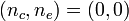$~(n_c,n_e) = (0,0)$ … ✓ ✓ Core-Envelope Structure with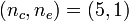$~(n_c,n_e) = (5,1)$ … ✓ ✓ Limiting Masses — Summary

### Stability:

 Solution Strategy Assuming Spherical Symmetry: Example Solutions:

### Dynamics:

 Spherical Collapse: Free Fall Homologous Collapse Collapse of Stellar Cores with n = 3 — investigation by Goldreich & Weber (1980, ApJ, 238, 991) Generalization to Arbitrary Polytropic Index

## Two-Dimensional Configurations

• Introduction

### Structure:

 Solution Strategies Axisymmetric Configurations Example Solutions: Maclaurin Spheroids Rotationally Flattened, Isothermal Structures Polytropic Tori: Papaloizou-Pringle (massless) Tori Self-gravitating Tori Infinitesimally Thin, Nonaxisymmetric Disk

## Three-Dimensional Configurations

• Introduction

### Structure:

 Solution Strategies Example Solutions: Ellipsoidal Figures of Equilibrium Compressible Analogs of Riemann Ellipsoids; see also Note to Hirschmann & Neilsen and Suggested Strategy by Joel